#### Option 3.2.2. Delta Accum

Select Delta Accum in the Calculation field on the Virtual tab of the Point Properties dialog box.

A Delta Accum point is an increasing representation of a source expression. A decrement in the source expression value is also considered to be an increase to the Delta Accum.

A Delta Accum point is particularly useful when used with a PLC to keep an incrementing tally as the PLC count increments and rolls over.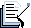Note: You can also use a Delta Accum point with a global virtual point. However, in most cases, the virtual point should be configured so that when it reaches the rollover level it emulates a PLC and rolls over to the initial value.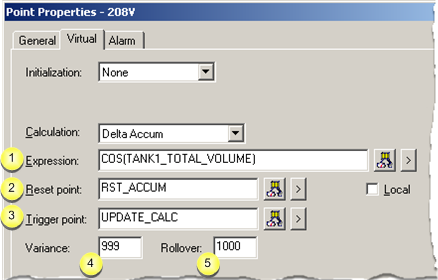Expression Reset point Trigger point Variance Rollover Delta Accum array configuration Guidelines for a Delta Accum point

 Expression field

Enter an expression that calculates the value of the Delta Accum point.

The expression will be monitored for transitions.

The expression may contain: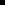One Point ID along withConstant values, operations, and functions.

Buttons to help with the equation are as follows.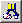Opens the Expression Editor. Displays a Popup menu to: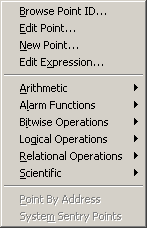Open: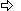The Select a Point browser.The Point Properties dialog box for a selected point.A New Point dialog box.The Expression Editor.Display an extended menu with the associated operations.
 Reset Point

Based on whether there is an entry in the Reset Point field, at runtime the Delta Accum point is reset as follows:

 When the trigger point is not defined If And Then An Initial Delta Accum Point Value is The Current value of the Expression is The Delta Accum point value is reset to the: Not defined Unavailable Zero Not defined Available Zero Defined Unavailable Initial value Defined Available Initial value When the trigger point is defined If Then An Initial Delta Accum Point Value is The Delta Accum point value is reset to the: Not defined Zero Defined Initial value

Note: Buttons to the right of the Reset Point and Trigger Point fields help with configuration.Opens the Select a Point browser to select an available point. Displays a Popup menu to: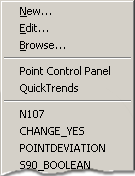Open:A New Point dialog box.The Point Properties dialog box for a selected point.The Select a Point browser.Select a recently selected point.
 Trigger point

(Optional) When the value of the Delta Accum point updates depends on whether or not you defined the Trigger point as follows:

 Trigger point The value of the Delta Accum point is updated whenever the Value of the: Defined Trigger point is updated. Not defined Source point in the Expression field is updated.

Buttons to help with the trigger point have the same functionality as the buttons to the right of the Reset Point field.

 Variance

If incoming data suddenly, for example, overloads the computer to the degree that the variance between the Delta Accum increment and the source becomes high enough that the Delta Accum misses a source rollover, the Delta Accum value ceases to be an accurate representation of the source activity.

The Variance field provides a way to alert the operator if this situation occurs.

When the difference between the new source value and the old source value exceeds the entry in the Variance field, the Delta Accum value does not increment. Instead a message is logged to the status log that this situation occurred.

Enter the maximum acceptable delta value in the Variance field that can be added to the Delta Accum point value.

If the delta value is greater than the Variance value, the:

1. New delta value is not added.

2. System logs the following message to the Status Log with Success status:

Variance value exceeded for accumulator point : <point_id>

 Rollover

(Optional)

Example

A Delta Accum point has a:Rollover value of 99.Current value of 98.

The previous source expression has a value of 97.

If a new value of +3 is received, the new delta accum is calculated as:

98+(100 - 97)+ 3= 104

If you do not specify a Rollover value, the size of the data type for the Delta Accum point determines the default Rollover value.

Example

If the source point has an INT point type, the maximum acceptable value for the Delta Accum point is 32767.

When the maximum value is exceeded the following message is logged to the Status Log with Success status:

Rollover occurred for accumulator point : <point_id>Note: If the source expression is a floating or Boolean point, rollover is not applied.

 Delta Accum Array Configuration

When you configure an array point as a Delta Accum point:The first element of the array adds the difference between the current and previous values of the source Point ID that you specify in the Expression field to the current value of the element.Each additional element of the array is used to store the delta accumulator value for a different subgroup of the array.

A selector point, which is defined in the Safety Point field, determines which subgroup element of the array is updated. The selector point must have a value from 1 to N-1, where N is the number of elements in the array.

Example

An array Delta Accum point tracks production counts for theTotal number of parts produced in a shift.Number of parts produced in each hour of the eight-hour shift.

The Delta Accum array point is called COUNTS with 9 Elements (one for the total shift count, and one for each hour of the shift). The elements are defined as follows:

 Element Description COUNTS Shift total production count COUNTS First hour production count COUNTS Second hour production count COUNTS Third hour production count COUNTS Fourth hour production count COUNTS Fifth hour production count COUNTS Sixth hour production count COUNTS Seventh hour production count COUNTS Eighth hour production count

A Safety Point called HOUR:Varies from 1 to 8, depending on the hour of the shift.Determines which subgroup element gets updated along with COUNTS. For example, if HOUR=4, COUNTS and COUNTS are updated.

If HOUR is less than 1 or greater than 8, no elements in the array are updated.

 Guidelines for a Delta Accum point

A Delta Accum point:Adds the difference between the current and previous values of the expression that you specify in the Expression field to the current value of the Delta Accum point.Is first updated after the first two expression updates are received.Continues to have Delta values added until a Reset Condition is reached.Is writable, i.e. you can use the Point Control Panel or a script to set the value, overwriting the accumulated value.Is always calculated as follows:

1. If the old source is less than the new source,

New Delta Accum = Old Delta Accum + (New source - old source)

1. If the source has rolled over so the new source is less than the old source,

New Delta Accum = Old Delta Accum + (Source rollover value + 1) - old source value + new source value

Example

Note: A rollover value, which is used in this example, is the maximum acceptable value for a source point. When the maximum value is incremented by 1 unit, the source point rolls over to 0.

A Delta Accum point has a value of 100 and a Rollover value of 1000.

1. The source changes from 100 to 999.

The new Delta Accum value=999.

999=100+(999-100)

1. The source next changes from 999 to 20. (The source point has rolled over.)

The new Delta Accum changes to 1022 based on the formula's calculation:

999+(1000 + 1) - 999 + 20 = 1022

 Step 3.2. Configure virtual calculations.